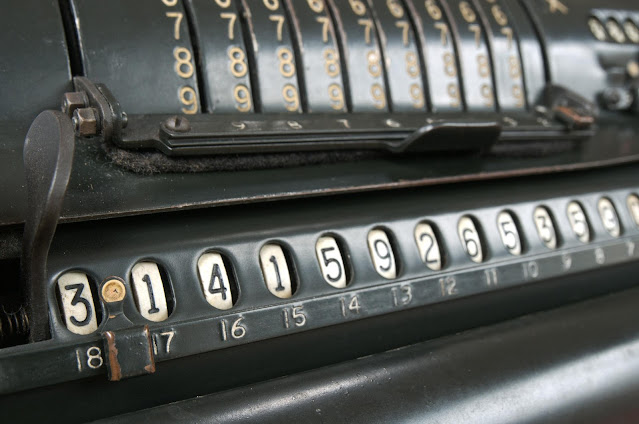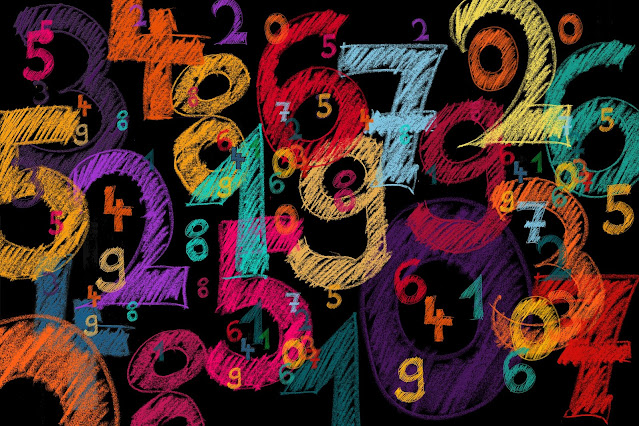## Posts

### Java Code to Partition an Array and return the maximized Sum of PairsGiven an integer array  nums  of  2n  integers, group these integers into  n  pairs  (a 1 , b 1 ), (a 2 , b 2 ), ..., (a n , b n )  such that the sum of  min(a i , b i )  for all  i  is  maximized . Return  the maximized sum This is a leetcode problem , which we will try to solve today.  On analyzing the problem we can understand that we need to split or partition the array into pairs of 2, and then take the minimum value from a pair, such that the sum of these minimum values is maximum.  Ideally, the value can be maximum only if we are taking bigger numbers, but we have to take minimum values from a pair of Integers. So, to work around this problem, we will sort the array first. This way, when we make pairs, we can ensure that the minimum value of a pair can come out to be a bigger number. We will then take the sum and return the same.  /**   * Group an array of 2n integers , into n pairs of two such that sum of min(ai , bi) for all i is maximized   * @param nums   * @retur

### Java code to Split a String into Maximum number of Balanced StringsWe are given a string which contains only characters 'R' and 'L'. A balanced string is one which has equal number of 'R' and 'L' characters. The problem statement is to find the maximum number of balanced strings which can be extracted from an input String. Here is a link to the leetcode problem, and we'll try to solve it today. /**   * Split the String into maximum number of Balanced Strings   *   * @author computengine.com   * @param s   * @return  */ public int balancedStringSplit(String s) { int countR = 0 , countL = 0 , count = 0 ; // loop through the input string for ( int i = 0 ; i < s . length ( ) ; i + + ) { // increment if 'R' found if ( s . charAt ( i ) = = 'R' ) countR + + ; // increment if 'L' found if ( s . charAt ( i ) = = 'L' ) countL + + ; // increment counter when Number of 'R' match Number of 'L', and reset

### Java code to find Count of Pairs in Integer Array with Absolute Difference KLet's solve a LeetCode problem using Java.  Count Number of Pairs With Absolute Difference K You can go through the problem and try to understand what needs to be done. Try to write your own code first. We have solved the problem and its here for your reference. /**  * Count Number of Pairs With Absolute Difference K  * @author computengine.com  * @param nums  * @param k  * @return  */ public int countKDifference ( int [ ] nums , int k ) { int count = 0 ; for ( int i = 0 ; i < nums . length ; i + + ) { for ( int j = i + 1 ; j < nums . length ; j + + ) { if ( Math . abs ( nums [ i ] - nums [ j ] ) = = k ) { count + + ; } } } return count ; } If you look closely, we have looped through the Integer Array, and compared two elements of the arrays and find the difference between them. Now the difference can be positive or negative but it has to be equal to the input K. So we've used Math.abs() function

### Selenium UI Automation - 15 different UI elements for Automation PracticeSelenium is a widely used Automation library used for UI automation. We are presenting the most comprehensive single HTML page which contains different types of UI elements which a user will encounter on websites, and this page can be used for Selenium Automation practice of the end user. The different types of fields covered in this practice page are: Radio button Dropdown Checkbox Switch to New Window Switch to New Tab Switch to Alert Web table Display and Hide Elements Date field Date and Time field Multi select field Browse button for windows file selection iFrame Email field Password field Radio Button Example Radio Button 1 Radio Button 2 Radio Button 3 Dropdown Example Select Dropdown Option 1 Dropdown Option 2 Dropdown Option 3 Checkbox Example Checkbox Option 1 Checkbox Option 1 Checkbox Option 1 Switch Window Example Open New Window

### 2 ways to convert a Number to Binary using Java (Custom function included)Converting an Integer to Binary format is a common problem which is taught in Computer Science. All computers read and understand Binary number format, which represents all characters as a combination of 0s and 1s. In order to convert an Integer to Binary, the method used is very simple. Since Binary Number system is based on 2 characters (0 and 1), we divide the Integer by 2 and store the result. We keep doing this till the number becomes 0. If we have to write a program in Java, how do we do that?  Let's check two ways to solve this problem in Java: 1. Using the Inbuilt function to convert to Binary We can use the inbuilt function toBinaryString which makes the process really easy. As per java documentation: String java.lang.Integer.toBinaryString(int i) Returns a string representation of the integer argument as anunsigned integer in base 2.  Let's look at the implementation: int number = 2256 ; System . out . println ( "Using inbuilt function:" + Integer . toB

### 3 Ways to find if a String contains a substring using Java (Bonus: Ignore spaces)So, you have a substring (or a word) which you have to find if its part of a sentence or not. We can do this in multiple ways in Java, depending upon which part of the sentence you are looking at, or if you want to include spaces in the search. Let's check out the ways we can do this: 1. Word can be anywhere in the sentence Or, the Substring can be anywhere in the String. If the word you are looking for can be in any part of the sentence, we can use contains() method.  As per java documentation: boolean java.lang.String.contains(CharSequence s) Returns true if and only if this string contains the specifiedsequence of char values. Let's check out the implementation. String baseString = "This is the String we are going to use" ; //any part of the string contains the substring System . out . println ( baseString . contains ( "we" ) ) ; System . out . println ( baseString . contains ( "hello" ) ) ; Output: true false 2. Word is at the start of the

### 6 ways to Concatenate Strings in Java (Bonus: Choose concatenation character)We need to deal with Strings in real time projects, and concatenation of Strings is a common and important functionality. There are multiple ways supported in Java to concatenate 2 or more Strings. Let's try to understand what are our options: 1. Using the '+' operator This is the easiest and the most commonly used option. We can simply use '+' between 2 Strings to concatenate them. String s1 = "Hello" ; String s2 = "World" ; System . out . println ( s1 + s2 ) ; Output: HelloWorld 2. Using concat() function Java has inbuilt function for concatenation. + operator is used for addition operations as well. If you want your code to be specific and readable, concat() function is an easy approach. String s1 = "Hello" ; String s2 = "World" ; System . out . println ( s1 . concat ( s2 ) ) ; Output: HelloWorld 3. Using StringBuilder class StringBuilder class has inbuilt methond append(), which we can use for concatenati